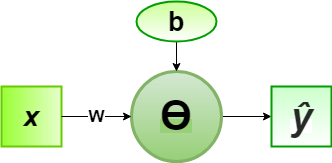Related Articles
Implementation of Perceptron Algorithm for NOT Logic Gate
• Last Updated : 08 Jun, 2020

In the field of Machine Learning, the Perceptron is a Supervised Learning Algorithm for binary classifiers. The Perceptron Model implements the following function:For a particular choice of the weight vectorand bias parameter, the model predicts outputfor the corresponding input vector.

NOT logical function truth table is of only 1-bit binary input (0 or 1), i.e, the input vectorand the corresponding output01
10

Now for the corresponding weight vectorof the input vector, the associated Perceptron Function can be defined as:For the implementation, considered weight parameter isand the bias parameter is.

Python Implementation:

 # importing Python libraryimport numpy as np  # define Unit Step Functiondef unitStep(v):    if v >= 0:        return 1    else:        return 0  # design Perceptron Modeldef perceptronModel(x, w, b):    v = np.dot(w, x) + b    y = unitStep(v)    return y  # NOT Logic Function# w = -1, b = 0.5def NOT_logicFunction(x):    w = -1    b = 0.5    return perceptronModel(x, w, b)  # testing the Perceptron Modeltest1 = np.array(1)test2 = np.array(0)  print("NOT({}) = {}".format(1, NOT_logicFunction(test1)))print("NOT({}) = {}".format(0, NOT_logicFunction(test2)))
Output:
NOT(1) = 0
NOT(0) = 1


Here, the model predicted output () for each of the test inputs are exactly matched with the NOT logic gate conventional output () according to the truth table.
Hence, it is verified that the perceptron algorithm for NOT logic gate is correctly implemented.My Personal Notes arrow_drop_up